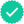Question

Discussion. Given an initial value problem
y
′ + p(t)y = q(t), y(t0) = y0 ,
the interval of validity is the largest interval that contain t0 and does not contain
any discontinuity for p(t) or q(t). The existence and uniqueness theorem guarantees
there is a unique solution on the interval of validity.
Problem: Without solving, determine the interval of validity for the initial value
problem
(t
2 − 9)y
′ + 2y = ln |20 − 4t| , y(4) = −3.

Expert SolutionKnowledge Booster••